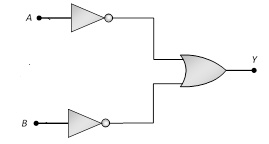# Digital Electronics MCQs For NEET

NEET  Physics is the scoring paper in the medical entrance examination. Here, you will discover the NEET Physics MCQ Questions for all Concepts as per the latest syllabus. Practice more on a regular basis with these NEET Physics objective questions on air pollution and improve your subject knowledge & problem-solving skills along with time management. NEET Physics Digital Electronics Multiple Choice Questions make you feel confident in answering the question in the exam & increases your scores to high.

## MCQs on Digital Electronics

1. The following truth table corresponds to the logic gate

 Input Output A B X 0 0 0 0 1 1 1 1 1 1 0 1

(a) NAND
(b) OR
(c) AND
(d) XOR

2. Boolean Algebra is essentially based on
(a) Truth
(b) Logic
(c) Symbol
(d) Numbers

3. The logic behind NOR gate is that it gives
(a) HIgh output when both the inputs are low
(b) Low output when both the inputs are low
(c) HIgh output when both the inputs are high
(d) None of the above

Answer: (a) HIgh output when both the inputs are low

4. A logic gate is an electronic circuit which
(a) Makes logic decisions
(b) Allows electrons flow only in one direction
(c) Woks binary algebra
(d) Alternates between 0 and 1 values

5. How many NAND gates are used to form an AND gate?
(a) 1
(b) 2
(c) 3
(d) 4

6. Which logic gate is represented by the following combination of logic gates?(a) OR
(b) NAND
(c) AND
(d) NOR

7. The output of OR gate is 1
(a) If both inputs are zero
(b) If either or both inputs are 1
(c) Only if both inputs are 1
(d) If either input is zero

Answer: (b) If either or both inputs are 1

8. Which of the following logic gate is a universal gate?
(a) OR
(b) NOT
(c) AND
(d) NOR

9. A gate in which all the inputs must be low to get a high output is
(a) NAND gate
(b) Inverter
(c) NOR gate
(d) AND gate